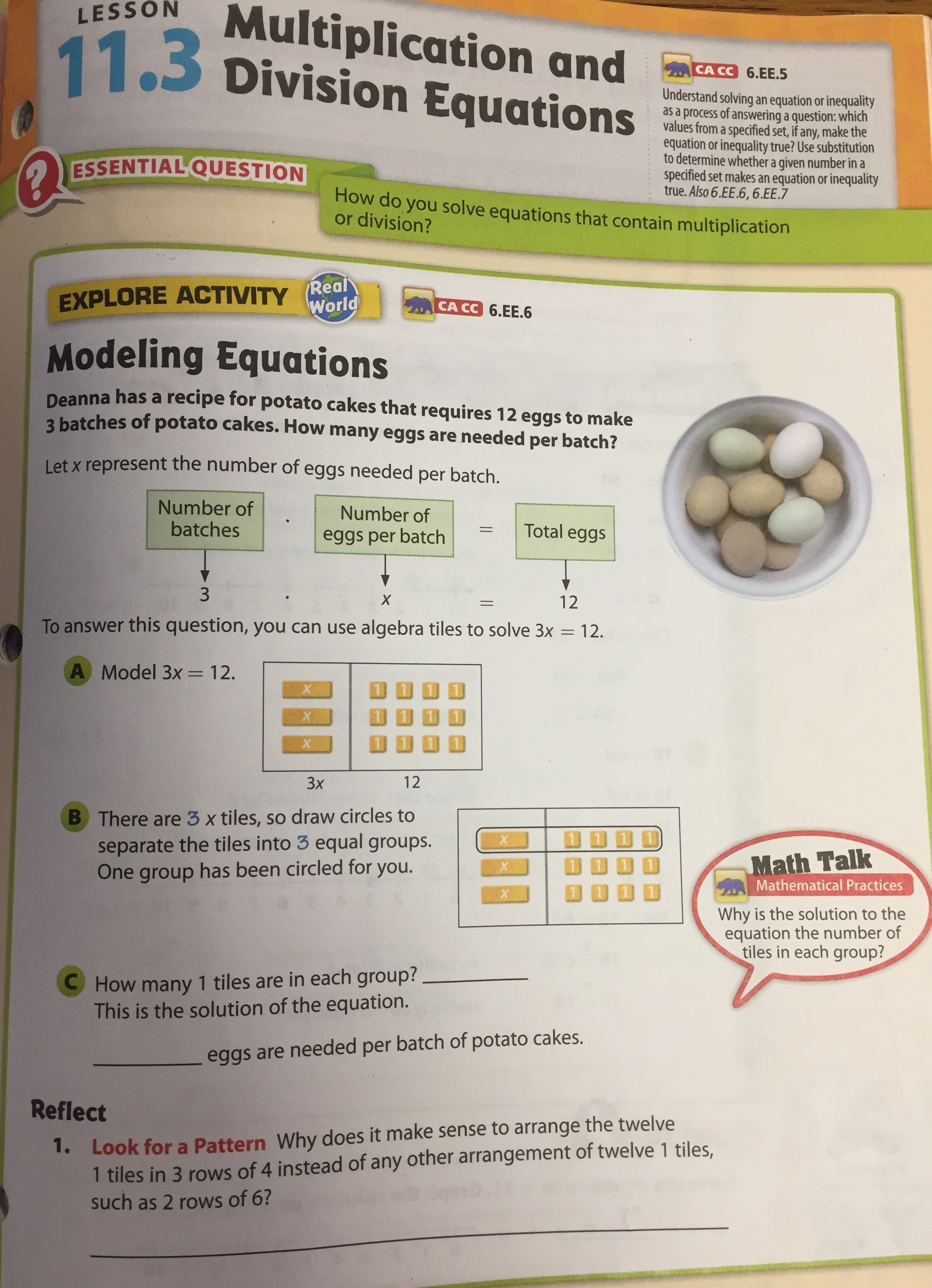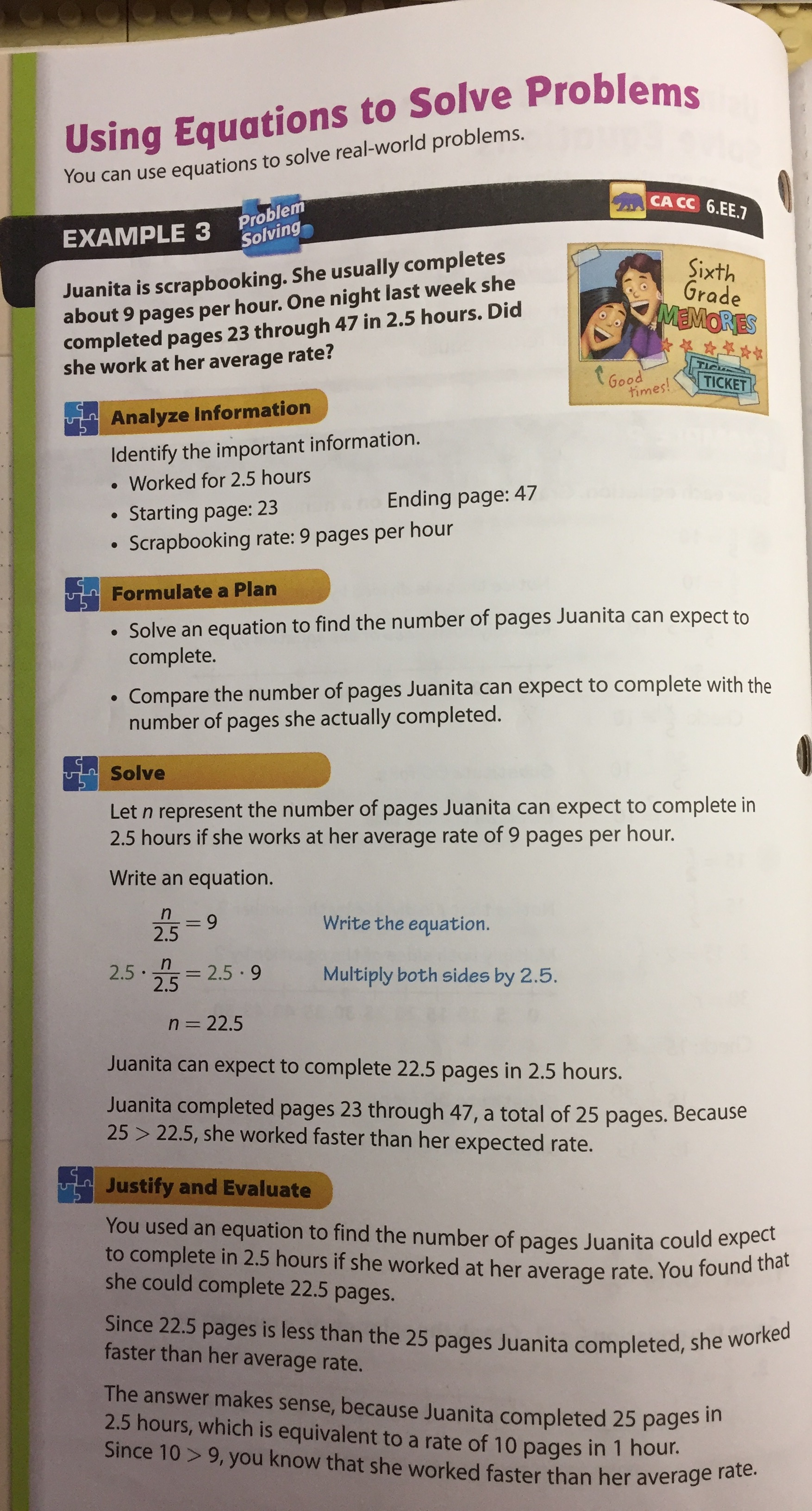# Seesaw 2: A 3-Act Lesson for 6th Grade Expressions and Equations

## My struggle:

I conduct a lot of lesson studies.  Much of the focus of that work is about putting student thinking at the center of the math classroom, not textbooks.  I work with teachers to create open, low-floor and high-ceiling lesson opportunities so all students have an opportunity to play and engage with math.

Usually, I’m pretty good at it.  But there are a few content strands that give me anxiety.  The 6th grade equation solving standards that ask students to reason about and solve one-variable equations have always stressed me out because I find it challenging to engage students in scenarios that get them using such simple equations to describe authentic problems.

Furthermore, the progressions state that “in addition to constructing and solving equations in one variable, students use equations in two variables to express relationships between two quantities that vary together….As they work with such equations students begin to develop a dynamic understanding of variables, an appreciation that they can stand for any number from some domain.”  (Emphasis mine.)

(Note:  You can find the 6.EE standards here and the progression document for expressions and equations here.)

Develop a dynamic understanding of variables?  That’s a tall order because much of the context I see in textbooks feels forced.  It’s challenging to create the necessary intellectual need to create, analyze, and appreciate the structure and usefulness of an equation.  This is often the case because textbooks spend pages and pages teaching (training?) kids to solve equations, not inviting them to create their own or to ponder the structure of equations given to them.

Here are two pages (out of about 12) from a textbook on 6th grade equations solving:It’s practically impossible to liberate student thinking from the clutches of this lesson structure.  It definitely won’t achieve the goals outlined in the progression asking students to develop a dynamic understanding of variables.

## My question:

How do we invite students to authentically engage with an equation in a way that invites students:

1. to appreciate how the structure of an equation models a context, and
2. to dive deeper in to the meaning of the relationships between variables?

Instead of teaching students how to use the properties of equality to solve “one-step” equations first (which is like using a bazooka to kill a cockroach by the way), I’m wondering if there’s a way to start the exploration of equation solving by inviting students to experience the dynamic relationship between variables first.

## My thinking:

I was inspired by a new 3-Act Math lesson by Graham Fletcher called Seesaw.  He created this lesson for early elementary students.  Here’s Act 1:

The key question becomes:  How many bricks will it take to balance the girl?

Act 2:  The weight of the girl (60 pounds) and the weight of each brick (5 pounds) is shared.  Students do some math.

And then Act 3 shows the big reveal:

It occurred to me that if we rearranged these Acts, we can create an opportunity for 6th graders to develop an equation and ponder possible solutions.  As students evaluate the reasonableness of solutions, they will continually be thinking about the context of the variables and the structure of the equation (and embodying Math Practice 2).

To elaborate, here’s a sketch of what I’m thinking for Act 1:

1. Show the Act 3 video as Act 1.
2. Ask students what they notice and wonder.
3. Students will notice that the weight of 12 bricks equals the weight of the girl.
4. They will wonder:  How much does the girl weigh?  How much does each brick weigh?

Now, that’s a pair of related variables bounded together by an equation!  Students can infer that g = 12b and analyze about the meaning of the variables and the coefficient 12 in the context of a problem.  The equation becomes the Act 2:  it’s the tool that allows them to describe possible solutions.

Furthermore, as students make estimations about either the weight of the girl or the weight of bricks, they will be solving the equation and creating their own solutions in the form of coordinate pairs.  These coordinate pairs can be graphed and further exploration about the relationship can be uncovered.  (For example, it appears to be proportional.)

After making estimates, students can try to determine a reasonable domain and range (not necessarily using those words).  For example, if each brick weighs one pound, the girl only weighs 12 pounds.  That doesn’t make contextual sense.  Therefore, “1” is not a reasonable input (domain) value for “b.”

After rich discussion about the equation (and it’s table and graph), Graham’s Act 2 images showing the weight of the girl can be used as the big reveal that out of many possible solutions, (5, 60) is the solution to this context.

## My Invitation:

Here’s a Desmos lesson I created:

https://teacher.desmos.com/activitybuilder/custom/59053e7beea92c4f782eb9a2

It’s not perfect.  It needs your help.  Feedback appreciated.

Also, what other resources do you use to get 6th (and 7th) grade students thinking about equations, equality, and the dynamic relationship between variables?  How do you put student thinking (and not the textbook) at the center of the discussion?

## 5 thoughts on “Seesaw 2: A 3-Act Lesson for 6th Grade Expressions and Equations”

1.lostinrecursion.wordpress.com I can show you what I did with my kids after Mike Fenton ran me across this page a while back. Basically, start with picture one and determine which shape is the heaviest and why….then add inequalities to determine which is heavier…LAST, very last, add number values that will prove the equation true.

•Thanks for sharing this resource! I’ve seen mobiles used to talk about equality, but not so much inequality. Love this structure and flow! Thank you!

2.Hi Chase,
Thanks for the post. I’m intrigued by your idea of using Act three and having students work back to different possibilities for Act One. I work in adult numeracy/high school equivalency. A very different student population, but there is a lot of overlap in content. I really like using visual patterns for some of this work with students. I have been inspired a lot by Fawn Nguyen’s work, especially on her visualpatterns.org site. I created a framework for hse teachers – Unit 8 is titled, Developing Algebraic Reasoning through Visual Patterns and explores some of our work. You can find Unit 8, as well as other supplemental materials I’ve gathered online here – http://www.collectedny.org/fpsubjects/unit-8/. Unit 8 has a visual pattern called The Arch Pattern, where students are given the first three figures and asked to consider various patterns. Once students have come up with one/several ways of determining how many squares (let’s say) there will be in the nth figure (either with equations or in statements, like, “Double the figure number and then add three more squares across the top”), I give students the number of squares and ask them which figure number would have that number of squares. I like it because students’ answers use inverse operations, not because that’s what you re supposed to do with equations, but because they are either physically (with manipulatives) or mentally laying out the number of squares and working backwards. They say things like, “Well, we need to take three squares and lay them across the top. Then we need to divide up the remaining tiles up into two even columns underneath. Since I know the height of the column is the figure number, it would be figure #__.”
Unit 7 of the framework focuses on equality, which is a challenge for many adult education students who understand the equal sign to mean “the answer”. There are a few great problems I love to use with students – Zip Zap Zowie, Fawn Nguyen’s ark problem, balance scales, etc. You can find unit 7 and all the supplemental resources we’ve gathered here – http://www.collectedny.org/fpsubjects/unit-7-equality/

•I’ll give in to these resources when I have more time! But thank you for sharing and thanks for the insights! Your adult students are fortunate to have such a teacher like you! Fawn’s stuff is amazing for helping all students think about patterns and numeric/algebraic expressions.

3.Planning to take this lesson outside and set it up live – an actual kid, actual paver bricks.

This site uses Akismet to reduce spam. Learn how your comment data is processed.